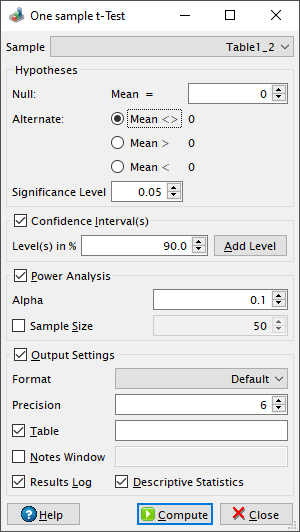One sample t-Test Dialog

This dialog is activated by selecting the One Sample t-Test... command from the Statistics -> Hypothesis Testing -> menu.

A t-test is any statistical hypothesis test in which the test statistic follows a Student's t-distribution under the null hypothesis. It is assumed that the data is normally distributed. The Wikipedia article on the Student's t-test makes for excellent reading on this topic.

Figure 5-85. One sample t-Test dialog.The one-sample form of a t-Test compares the mean (m) of a population to a mtest value specified in the null hypothesis. By default QtiPlot performs a two-tailed test, the default alternate hypothesis being that the mean of the population and the mtest values are different (m <> mtest). It is also possible to perform an upper-tailed test by choosing the alternate hypothesis that m > mtest or a lower-tailed test (m < mtest).

This test uses the statistic t = N1/2(m-mtest)/SD, where N is the size of the population and SD is the standard deviation, SD = [Σ(xi - m)2/(N-1)]1/2.

The test statistic is used to compute a probability (P value). For a lower-tailed test the probability is calculated using the formula p = tcdf(t, DoF), where the tcdf function calculates the lower tail of the cumulative distribution function for the Student's t-distribution with DoF = N-1 degrees of freedom. For an upper-tailed test, the probability is calculated using the formula p = 1-tcdf(t, DoF). For a two-tailed test the probability is calculated using the formulas p = 2tcdf(t, DoF) if tcdf(t, DoF) < 0.5 and p = 2[1-tcdf(t, DoF)] otherwise.

The null hypothesis is rejected if the calculated probability is lower than the value of the Significance Level.

If the Confidence Interval(s) box is checked QtiPlot also computes a confidence interval (Lower Limit and Upper Limit) for each user defined level.